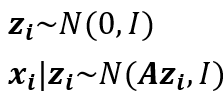# Get error when implementing custom model

#1

Hi, sorry to bother you again.

I want to implement the model (sub module of a big one) as the image showed below.When I run the model below, I got a error said `RuntimeError: The size of tensor a (100) must match the size of tensor b (4) at non-singleton dimension 1`.

It seems there are bugs in my code. Please give me some suggestions.

Thanks.

``````N,D,K= 100,4,2
pyro.clear_param_store()
def model():

A = pyro.param('A',torch.rand(D,K))

with pyro.plate('sample_dim',N,) as idxN:
with pyro.plate('components',K,) as idx:
z=pyro.sample('z',dist.Normal(0,1))

print(z.shape) #torch.Size([2, 100])
print(A.shape) #torch.Size([4, 2])
temp = torch.matmul(A,z)
print(temp.shape) #torch.Size([4, 100])

with pyro.plate('sample_dim_1',N,) as idxN:
x = pyro.sample('x',dist.Normal(temp[:,idxN],t.diag(t.ones(D))))
return
``````

#2

`pyro.plate('sample_dim_1',N,) as idxN` will give you a tensor of indices of size `N`. so `temp[:, idxN]` has shape `(4, 100)` and `t.diag(t.ones(D))` has shape `(4,4)`

#3

Thanks!

It’s a stupid bug…